C Program to Swap Two Strings

In this tutorial you will learn about the C Program to Swap Two Strings and its application with practical example.

C Program to Swap Two Strings

In this tutorial, we will learn to create a C program that will Swap Two Strings in C programming.

Prerequisites

Before starting with this tutorial we assume that you are best aware of the following C programming topics:

• Operators in C Programming.
• Basic Input and Output function in C Programming.
• Basic C programming.
• Conditional statements in C programming.
• String functions of c programming.

Program to Swap Two Strings

As we all know the String is a collection of character data types. In strings, only one variable is declared which can store multiple values. First, we will take the input string from the user. Then we will Swap Two Strings using the third variable that will help to swap.

With the help of this program, we can Swap Two Strings.

Output:-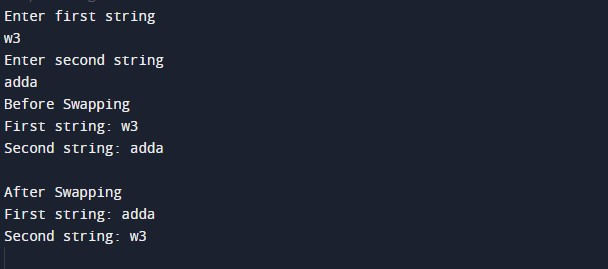In the above program, we have first initialized the required variable.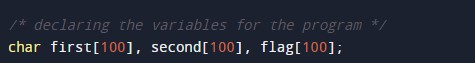• first = it will hold the string value.
• second = it will hold the string value.
• flag = it will hold the integer value.

Taking the input string 1 from the user.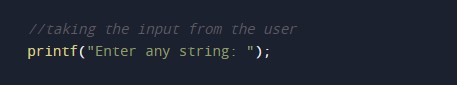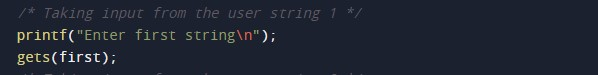Taking the input string 2 from the user.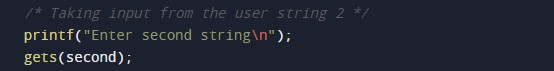Print string before the swap.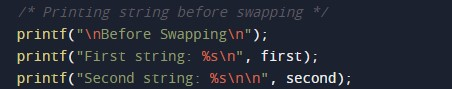Swapping the strings,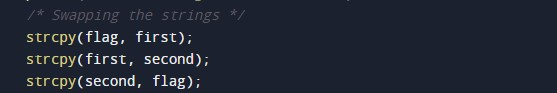Printing the output.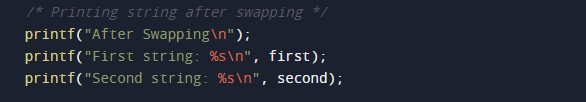In this tutorial we have learn about the C Program to Swap Two Strings and its application with practical example. I hope you will like this tutorial.